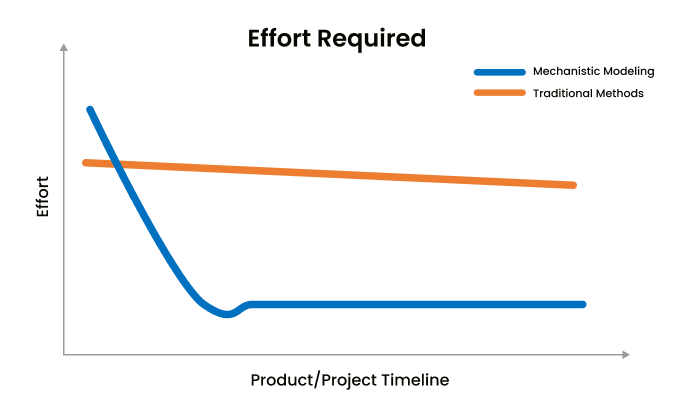# Mechanistic Approach in Engineering Problem Solving

## Mechanistic Approach in Engineering Problem Solving

Engineering problems can be highly complex, and while some simpler ones may be resolved through analytical calculations, the advent of computational technology has given rise to Finite Element Modeling (FEM), which utilizes numerical calculations to solve complex problems that were previously unsolvable through basic mathematical computations.

Engineering problems can be highly complex, and while some simpler ones may be resolved through analytical calculations, the advent of computational technology has given rise to Finite Element Modeling (FEM), which utilizes numerical calculations to solve complex problems that were previously unsolvable through basic mathematical computations.

Mechanistic modeling is a fusion of modern data science techniques and mathematical science employed to tackle complex engineering problems.

The following illustration provides a clearer explanation:To build a Mechanistic model, the following steps should be taken:

 Step 1 Identify the problem that needs to be solved. Step 2 Perform a root cause analysis to determine the underlying factors contributing to the problem. Step 3 Identify the major influencing factors among the contributing factors. Step 4 Extract mechanistic features in the form of a dataset corresponding to the major influencing factors. Step 5 Perform exploratory data analysis to gain insights into the data. Step 6 Perform feature engineering to prepare the data for machine learning models. Step 7 Build and evaluate appropriate machine learning models using the features extracted with underlying physics in consideration.

Since machine learning models are built with features extracted from underlying physics, the reliability of a mechanistic model is higher than that of a plain statistical model. The study of system characterization can be classified into three categories: single system characterization, homogeneous multi-system characterization, and heterogeneous multi-system characterization.

The major advantage of mechanistic modeling is its ability to capture characterization from different forms of inputs, such as design inputs, process inputs, and manual inputs, in engineering problems. In a Finite Element Analysis (FEA) analysis, it is nearly impossible to study the combined effect of all kinds of inputs. However, mechanistic modeling can capture this effect in the form of a data model.Another advantage of mechanistic modeling is that, unlike traditional methods, the scientific efforts required are concentrated primarily in the initial stages of the product or project. As the project progresses, the scientific effort required significantly reduces. In contrast, traditional methods require constant effort throughout the product or project lifetime. Thus, mechanistic modeling is a powerful approach for solving complex engineering problems.